# On the Behavior of 2d Transformations in Internet Explorer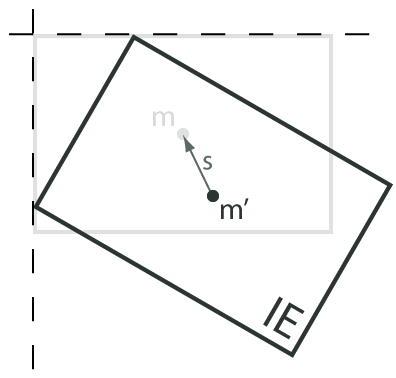In my work on the box2d and web worker modules in gwtns, I’ve needed the ability to put things up on the screen. To really make sure I was doing the word “overkill” justice, I decided to use my old TransformedElement module for that purpose. This has given me the opportunity to go back and ruminate over my original implementation.

Though it was only a few months ago, I originally took it on as more of a way to get to know the GWT deferred binding system than to write the perfect transformation library, and I wrote it in definite haste. I would probably not structure it the same way a second time. It’s not bad, but the differences between the underlying IE and CSS3 implementations are enough to make the unifying API a little less straightforward than it should be.

What was terrible, though, was the speed of the Internet Explorer version. The trial and error process to get IE’s matrix filter fully operational was annoying enough that I didn’t spend much longer on it than I had to. It helped that I only needed to position elements, not animate them, but that’s no longer true. As a result, I took a day last week to profile and revamp the IE implementation. The performance is actually now on par with the Firefox version, since the majority of execution time is taken up by DOM access.

But I’ll write more about the changes I made in my next post. For now, I really want to address how to get Internet Explorer transforming elements in almost the exact same way as all the “modern” browsers that support the CSS 2D Transforms (draft) specification. I’ve been surprised at the lack of support for IE as plugins and tools have started popping up to let authors easily transform elements. In fact, many explicitly state that IE support seems possible, but it hasn’t been implemented yet due to the pain of figuring out the different format.

But there’s no reason to repeat ourselves as a community. Supporting IE is actually pretty straightforward, you just have to be a little tricky to get around a few problems. With this done—if you’re willing to forsake Firefox users prior to 3.5 and Opera users prior to 10.5—essentially every browser in use is capable of applying geometric transformations to HTML elements. In writing this, I’m hoping that I can at least set a baseline of understanding of how transformations work in IE. That way, those that don’t want to figure it out from scratch won’t need to, and those that do will be able to concentrate on creating tools more elegant and sophisticated than I have here.

### A few caveats

• Unless otherwise stated, this post will be strictly about two dimensional transformations. So when I talk about “affine transformations” or “translations,” just read “2d affine transformations” or “2d translations.”
• I’m going to assume some familiarity with basic linear algebra and transforms. I’m hoping, though, that I can provide enough context so that the proper Wikipedia or MathWorld search will be clear even for unfamiliar concepts. Please let me know if and where I confuse or gloss over an important detail.
• This will only cover support for the equivalent of the matrix transformation function. i.e. transform: matrix(a, b, c, d, e, f); rather than the list of transformation functions: transform: rotate(<angle>) scale(<number>) ...;. I’m primarily interested in transforms through scripting—so I concatenate transformations into an internal matrix representation—but it’s trivial to find the matrix form of any list of transformations. This has implications for animation, but that will have to fall outside the scope of this post.
• All listed code was tested only in Internet Explorer 8. The Matrix Filter was added in IE5.5, but the accessor syntax was slightly changed in the latest version to better comply with the standard way of extending CSS. The syntax changes should be trivial, but layout changes are probably not. If you work it out, please let me know so I can put up a link.
• Finally, if all of this isn’t your cup of tea, I’ll have the final code posted next. Don’t worry; it’ll be JavaScript.

### UA Background

The type of transform we’re interested in is called an affine transform, which describes most of the ways one would want to move or change an object: scaling it, rotating it, shearing it, translating it, etc. There used to be no standard way to transform DOM elements, but a few years ago Apple started pushing for their format (which started life on the iPhone) to be adopted. From there, it spread to the desktop version of Safari and then eventually to Firefox, Chrome, and Opera. It’s now close to being finalized.

But it turns out that Internet Explorer has been well ahead of the pack for years, supporting the transformation of elements through its CSS “filter” extension since at least 2000. A quick Google search will actually find mention of it all over the place in old DHTML tutorials, but I can’t think of any time I’ve seen it in the wild. Like the existence of Flashblock, the fact that spinning webpages aren’t more widespread is probably evidence of divine providence and existing barriers shouldn’t be trifled with. But in the end, I prefer tools that will cheerfully help you shoot yourself in the foot (or your users in the eyes). We’re just going to have to rely on collective good taste.

A still forthcoming blog post better compares the results of IE’s matrix transform filter to the results of current CSS3 implementations, but, in theory, they are close to identical in what they support.

### Math Digression: Linear Transformations

An affine transformation is actually a combination of a linear transformation and a translation. In our case, the linear transformation takes linear combinations of a point’s x and y coordinates to map them to new coordinates. In other words, for point x$\mathbf{x} = \begin{bmatrix}x \\ y \end{bmatrix}$

linear transformation T produces the new point$\mathbf{T}(\mathbf{x})=\begin{bmatrix}ax + cy \\ bx + dy \end{bmatrix}$

or, in matrix form:$\mathbf{T}(\mathbf{x})=\begin{bmatrix}a & c \\ b & d \end{bmatrix}\begin{bmatrix}x \\ y \end{bmatrix} = \begin{bmatrix}ax + cy \\ bx + dy \end{bmatrix}$

By using specific values for the entries of the transformation matrix, here represented as a through d, a single linear transform can express a rotation, a scale, a shear, or even an ordered sequence of these operations combined. It can be very illuminating to work out what these specific matrices are for yourself, but as a simple example, an expansion by a factor of two would be represented like this$\mathbf{S}_2(\mathbf{x})=\begin{bmatrix}2 & 0 \\ 0 & 2 \end{bmatrix}\begin{bmatrix}x \\ y \end{bmatrix} = \begin{bmatrix}2x + 0y \\ 0x + 2y \end{bmatrix} = \begin{bmatrix}2x \\ 2y \end{bmatrix}$

This transformation would map every point to a new point at twice the distance from the origin, except the origin itself.

In fact, no linear transformation can move the origin. Rotations provide another easy example: no matter how many times a wheel is rotated, there is no rotation that will change the center of the wheel; that point is fixed. If we want to be more precise:$\mathbf{T}(\mathbf{0})=\mathbf{T}(\begin{bmatrix}0 \\ 0 \end{bmatrix})=\begin{bmatrix}a0 + c0 \\ b0 + d0 \end{bmatrix}=\begin{bmatrix}0 \\ 0 \end{bmatrix}=\mathbf{0}$

At the origin, the values of a, b, c, and d are irrelevant; a linear transformation always maps the origin to itself.

### Further Math Digression: Translations

Translations are what allow objects to move around without distortion. There are a few different ways to think of a translation, but the end effect is that all points (including the origin) are shifted in the same direction by the same amount. As noted, there is no way to do this with a simple linear transformation matrix because (among other things) there is no way for it to move the origin.

We’d really like to express the full affine transform as a matrix, though. Why would this be desirable? For our purposes, the main benefit is transform concatenation. Since matrix multiplication is associative, a chain of transformations applied consecutively to an object is equivalent to the application of the single product of each transformation’s matrix. Instead of an unbounded list of transformations, each requiring yet more operations to find an end result, each transformation can be multiplied into an intermediate matrix, requiring no more storage than the entries in that matrix.

If we can represent a linear transformation and a translation in a single matrix, more sophisticated behavior also becomes possible. For example, objects would be able to rotate about any specific point rather than always rotating about the origin. Our job also becomes easier; rather than dealing with a bunch of bookkeeping to keep two separate data structures geometrically synchronized, we keep only one structure (a matrix) and one very simple operation (multiplication). The problem remains, though, that matrices can only represent linear transformations, and a translation is not a linear transformation.

We cheat this by augmenting the matrix used so that we are now applying a linear transformation to a 2d plane in a 3d space. By convention, we add a z-coordinate of 1 to all of our 2d points, which guarantees we always have a non-zero coordinate with which we can play. Since the origin is no longer the actual origin (it’s now at (0, 0, 1)), we can shift it. We are actually shearing in 3d space, but when we discard the extraneous z-coordinates and look again at just our original 2d points, it appears as if a translation was applied.

If that’s not your kind of explanation, maybe the arithmetic will be a little clearer. Again, we augment our points so they are now in 3-space, and our matrix needs to likewise be upgraded to a 3×3 version:$\begin{bmatrix}a & c & e \\ b & d & f \\ 0 & 0 & 1 \end{bmatrix}\begin{bmatrix}x \\ y \\ 1 \end{bmatrix} = \begin{bmatrix}ax + cy + e \\ bx + dy + f \\ 1 \end{bmatrix}$

Comparing this result to the linear-transformation matrix multiplication in the previous section, it should be easy to see both the linear transform and the added translation at work. The e and the f entries, since they will always be multiplied by 1, move all points e-units horizontally and f-units vertically.

I purposefully left the bottom row of that matrix as [0, 0, 1]. Some really cool and interesting things can be done by altering the entries there, but without being careful with them, some sticky mathematical situations can arise (especially with invertibility). All the current browsers avoid this (in 2d land, at least) by only accepting transformations specified by the top 2×3 entries of the transformation matrix.$\begin{bmatrix}a & c & e \\ b & d & f \end{bmatrix}\begin{bmatrix}x \\ y \\ 1 \end{bmatrix} = \begin{bmatrix}ax + cy + e \\ bx + dy + f \end{bmatrix}$

The form is more limited, but for our goals it is sufficient.

This briefest of reviews will have to do for now. If you’d like to learn more, I suggest basically anything by Jim Blinn (in particular, his A Trip Down the Graphics Pipeline and its treatment of homogeneous coordinates). For more immediate gratification, Wikipedia does a pretty good job here.

### And Back to IE

As mentioned earlier, Internet Explorer accepts a transformation matrix through its filter extension to CSS; in Javascript you might set the filter from a matrix like this:

 element.style.filter="progid:DXImageTransform.Microsoft.Matrix(M11=a, M12=c, M21=b, M22=d, Dx=e, Dy=f, SizingMethod = sMethod)"; 

where a, b, c, and d still represent a linear transformation, and e and f represent a translation. These could be hardcoded values or dynamic ones, varying due to time and user input.

The SizingMethod property tells IE how to deal with elements that exceed their original bounds when transformed. If SizingMethod is left at its default value—the string “clip to original”—everything works and the element is transformed correctly. However, the rendering of it usually leaves something to be desired. If it was rotated or translated or scaled in a way that takes part of it outside of its original bounds, that part will be clipped. For example, a simple translation of 35 pixels to the right yields: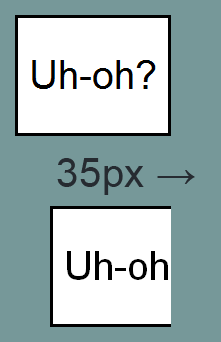So the default SizingMethod ends up being not very useful, but this isn’t unexpected given “clip to original;” everything seems sane.

The other possible value for SizingMethod is “auto expand,” which allows the transformed element to take up as much room as it needs (notably, without changing layout, just like the CSS3 rec). This would seem like the key, but it comes with a catch: if SizingMethod is set to “auto expand,” then all translation values will simply be ignored: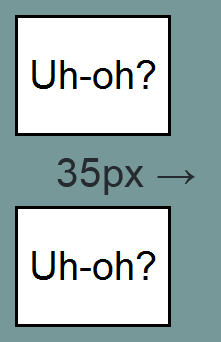I have absolutely no idea what the reasoning was here, or how anyone thought that this behavior would be functionally useful. The MSDN documentation states it so matter of factly that I feel like I must be missing something (or taking crazy pills), but I haven’t run across anything that actually explains the behavior. Fortunately, there are ways to work around this problem. Mostly.

### Workaround 1

As other people have also realized, the solution is to re-split the desired affine transformation. An augmented matrix is still used throughout the transformation process to allow transforms to be combined, but when it comes time to write the transform to an element’s style, the linear transformation and the translation are separated once again. The matrix filter (and “auto expand”) is used for the linear portion of the transformation. Since the translation is useless there, the element is instead translated as any other element would be: by altering its ‘left’ and ‘top’ attributes.

There are a few minor regressions inherent to this approach. First, translations now alter layout: as an element is translated around the page, any elements positioned relative to it will also have their positions altered. Currently I work around this by either only transforming absolutely positioned elements, or by wrapping an absolutely positioned element with a relatively positioned one, set to the same original dimensions. This has the effect of keeping the rest of the layout stable as the transformed element moves at will. It’s not pretty, but it works.

The other minor problem is that elements are now limited to integer pixel positioning instead of the nice floating point values they could use before. Smart rounding can mitigate the effect, but some object jittering will always be present, especially in slow movements or with small elements.

But there’s a more fundamental hurdle. As stated earlier, by its very nature a linear transformation leaves the origin of an element unaffected. A compliant CSS3 transform with no translation does this: origins stay put. IE is different; it transforms an element, calculates a bounding box for it, and then places that box’s top left corner at the specified ‘top’ and ‘left’ coordinates, origin be damned. Hasty info-graphic ahoy: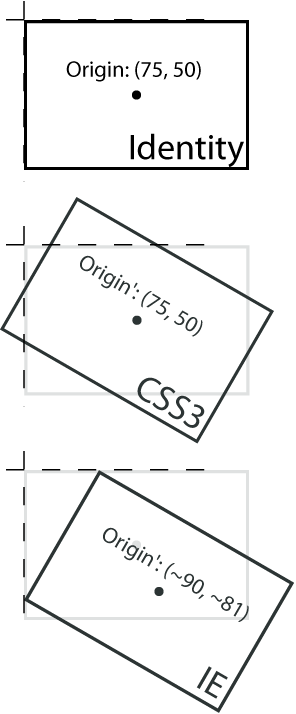The default origin for both the CSS3 Transforms spec and IE is found at the center of an element (given here in screen coordinates). When a pure linear transform is applied to it (in this case a rotation of 30 degrees), CSS3 keeps the origin fixed. IE’s bounding box routine will instead ensure that an element’s left-most point is at its ‘left’ value, and its top-most point is at its ‘top’ value. While this may seem straightforward in its description, in practice you just end up with bouncy boxes. No point is fixed. This becomes clear when you see it in action: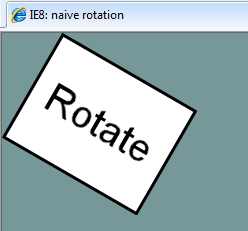Again, I have no idea how someone implemented this, tested it, and thought it useful, but not all things are revealed to me.

(In a rotation, the origin’s movement can be described by two catenary curves. That is cool. But not useful.)

### Workaround 2

Since IE keeps no point fixed under a linear transformation, if a translation is then just naively applied, an element’s final position will have shifted not by the translation value but by (translation + (some origin shift)). We need to compute that shift and remove it every time.

Take horizontal positioning first: Let x be the ‘left’ position of the original (untransformed) element, let w be its original width. The element’s horizontal midpoint is at$m_x = x + \frac{w}{2}$

Finding the midpoint of the bounding box can have serious impacts on performance, but we will only concern ourselves with the theory for now and assume we already know its dimensions. What follows wouldn’t really be a proof, relying on an appeal to intuition with one visual example, but for one simple fact: a rectangle under a 2d linear transform shares its center with its minimum axis-aligned bounding box. The proof of this is straightforward, so I’ll leave it as an exercise for the reader.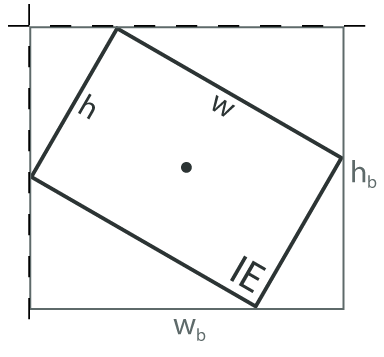Let wb be the width of the bounding box of the transformed element. Note that wb is not a transformed version of the vector (w, 0). This is obvious under a 90° rotation, as wb would have length 0, while the bounding box would actually have a width equal to the height of the original element.

Since it shares its center with its bounding box, and we’ve assumed that we know the width of that box, the transformed element’s midpoint has moved to$m_x^\prime= x + \frac{w_b}{2}$

Since we want the bounding box (and thus the transformed element) to be horizontally centered at mx, not m’x, we subtract the difference of the two from the translation value before we apply it to the element. If we call the horizontal shift sx$s_x= m_x^\prime - m_x=\frac{1}{2}(w_b-w)$

The vertical shift is found similarly.$s_y= m_y^\prime - m_y=\frac{1}{2}(h_b-h)$In JavaScript, the shift would then be removed when applying the translation to the element:

element.style.left = x + e - sx + 'px'; element.style.top = y + f - sy + 'px';

Finally, while I’m not going to go in depth on support for changing the origin, it’s just an additional adjustment, this time by the transformed difference between the center of the original element and the requested new origin. If you’d like to take a look at my solution, it’s located here. The code is somewhat obfuscated for performance, but it shouldn’t take long to figure out (yes, that’s Java. It’s still weird for me too).

### Next

That’s it for now. If you just want the code, or you’re dying to know how the replacement of two lines of code with twelve resulted in an order of magnitude speedup, stay tuned for the next post, entitled, “The DOM,” or, “The API Only a Mother Could Love.”